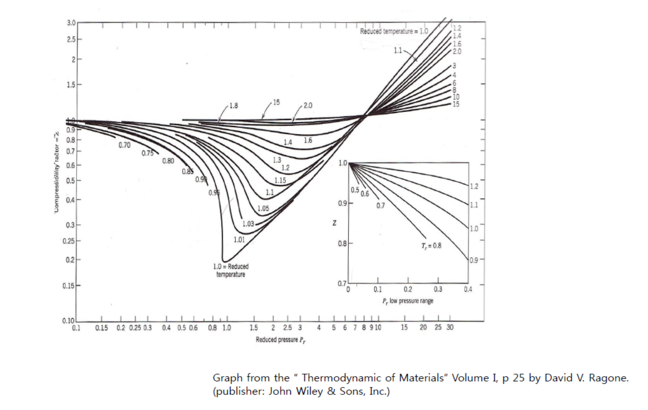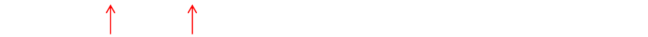## Want to keep learning?

This content is taken from the Hanyang University's online course, Thermodynamics in Energy Engineering. Join the course to learn more.
2.12

# Equation of state for non-ideal gases

• The P-V-T relationship for real gas needs modification of ideal gas laws.
1) Compressibility factor
The compressibility factor Z is a correction term to the ideal gas laws.
PV=ZRT, z : compressibility factor
- Z can be estimated using the concept of “corresponding states”.
It states that gases behave similarly with respect to non-ideality;
that is, they have the same Z value when they are in corresponding
states relative to their respective critical pressure and critical
temperature.

- Thus, Z can be determined by knowing reduced pressure P$$r$$, and
reduced temperature T$$r$$.

- The Z values are tabulated (graphed) as a function of P$$r$$, T$$r$$, thus Z
depends on P, T.

$$Pr=\frac{P}{Pc}, Tr=\frac{T}{Tc}$$

where P, and T are the actual pressure and temperature and Pc
and Tc are the critical values.2) Van der Waals (vdw) equation of state
From ideal gas law, corrections in
① Volume
Real gas has finite volume (ideal gas = volume-less)
→ subtract the gas volume from the total volume.

② Another consideration
Particles are interacting each other. This correction should be     considered in the pressure term.

$$(P$$+$$\frac {a}{V^2})$$   $$(V$$−$$b)$$=$$RT$$ $$per$$ $$mole$$